# How to write recursive LAMBDA function in Excel with examples

The aim of this tutorial is to explain the concept of a recursive function and demonstrate the generic approach to the creation of recursive Lambdas in Excel. We will explore every aspect in depth to make it easy for you to follow and reproduce in your worksheets.

With the introduction of the LAMBDA function, recursive computations in Excel have become available to anyone, not just VBA programmers. To put it simply, you can now construct formulas that behave like a programming language and allow you to achieve so much, with so little, so fast :)

Please keep in mind that the examples discussed in this tutorial imply that you already know LAMBDA's syntax and basic uses. If not, it stands to reason to start with the essentials: How to write and use LAMBDA in Excel.

## Recursive LAMBDA function

To make sure that everyone is on the same page, let's first determine what a recursive function is.

In computer science, recursion is a method of solving a problem in which a function calls itself directly or indirectly. Such a function is called recursive. Basically, a recursive function works by iteration and finds a solution to a bigger problem by solving smaller instances of the same problem.

Currently, LAMBDA is the only Excel function that supports recursion, enabling you to create compact and elegant solutions for complex problems with no coding.

In VBA, recursion is generally done using a For… Next or Do… While loop. LAMBDA typically relies on the IF function to test a Boolean condition and recurse if the condition is either TRUE or FALSE.

Here's the structure of a recursive LAMBDA function it its simplest form:

=LAMBDA(x, y, …,       'declare parameters

IF(logical_test,    'test the condition

MyLambda(),      'recurse if the condition evaluates to TRUE

value_if_false)  'exit if the condition evaluates to FALSE

)

The key point is to stop recursive calls from continuing forever. For this, you should provide the ending case (also called the halting case, or base case). If no exit point is provided, a formula will keep iterating until your computer crashes, just kidding of course, it will throw a #NUM! error.

Compared to non-recursive functions, recursive Lambdas are more difficult to write, test and debug. It resembles the good old chicken and egg riddle - for a function to work correctly, it must call itself; to call itself, the function must work correctly :)

## Example of recursive LAMBDA to remove unwanted characters

When importing data from external sources, rubbish characters may often sneak in, and you need to find a way to clean your data somehow.

The Replace All feature can remove all occurrences of a given character by replacing them with nothing, but it can only deal with one character at a time.

A lot faster and more convenient will be to list all unwanted characters in some cell and eliminate them in one fell swoop using a formula. A recursive LAMBDA is exactly what you need:

`=LAMBDA(data, chars, IF(chars<>"", RemoveChars(SUBSTITUTE(data, LEFT(chars, 1), ""), RIGHT(chars, LEN(chars) -1)), data))`

Our custom Lambda function is named RemoveChars and it requires two input parameters:

• Data - a cell or a range of cells to be cleaned.
• Chars - the unwanted characters to remove. Can be provided in the form of a text string or a cell reference. In a cell, the characters should be listed without spaces, unless you want to eradicate spaces too.

At a high level, here's what the function does:

The RemoveChars function cycles through the exclusion list (chars) and purges one character at a time. Before each recursive call, the IF function evaluates the remaining chars. If the string is not empty (chars<>""), the function calls itself. As soon as the last character has been handled, the iteration process finishes - the formula returns data it its current form and exits.

The reverse logic will also work: if the chars string is empty (chars=""), then return the present data and exit; otherwise, call the RemoveChars function:

`=LAMBDA(data, chars, IF(chars="", data, RemoveChars(SUBSTITUTE(data, LEFT(chars, 1), ""), RIGHT(chars, LEN(chars) -1))))`

Whichever approach you choose, the result will be exactly the same: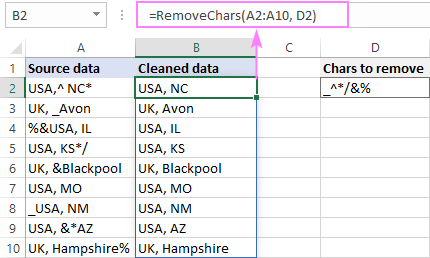## How to write recursive LAMBDA in Excel

I'd like to start with a disclaimer :) There is no documented way of building recursive Lambdas in Excel, which is explicable given that the function is brand-new. I will share my way, which may or may not be helpful to you.

### Create the core formula

Generally, you begin with writing the core formula(s) that emulate the desired behavior of your LAMBDA function. In our case, the ultimate goal is to replace specific characters with nothing, and Excel already has an ideal instrument for this - the SUBSTITUTE function:

SUBSTITUTE(text, old_text, new_text, [instance_num])

To SUBSTITUTE, we need to supply:

• Text - the text in which to substitute characters. In our case, it's a text string in A2.
• Old_text - the character to be replaced. We need to check every single character in D2, and it stands to reason to begin with the leftmost one. The LEFT function can easily fetch it for us:

`LEFT(D2, 1)`

• New_text - the character to replace old_text with. Obviously, it's an empty string ("").
• Instance_num is optional and is not needed in our case, so it's omitted.

As the result, our core formula takes this form:

`=SUBSTITUTE(A2, LEFT(D2, 1), "")`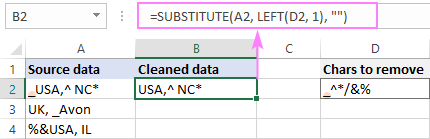Because SUBSTITUTE can only do one replacement at a time, it has to be executed as many times as there are characters on the exclusion list in D2. The question is - how do we force it to handle the next character? And here's the answer:

With each iteration, we'll strip off one character from the left, i.e. the character that has already been looked at. The RIGHT function in combination with LEN can easily do that:

`=RIGHT(D2, LEN(D2) -1)`

Please pay attention that each subsequent SUBSTITUTE uses the result from the previous SUBSTITUTE as the text argument, i.e. it makes the replacement not in the original string (A2), but in the string returned by the previous SUBSTITUTE functions (B2):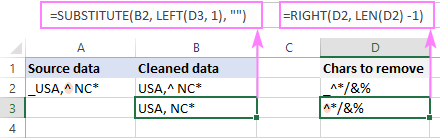### Convert the core formula to a LAMBDA function

As you remember, our custom function is supposed to be named RemoveChars, and it will have 2 parameters: data and chars.

Your job is to instruct the function on how to calculate each parameter:

• Data ­- the string in which to substitute characters. It is provided by the SUBSTITUTE formula.
• Chars - the characters to remove. It is provided by the RIGHT formula.

What you do is simply place the two formulas discussed above inside of the RemoveChars function separating them with commas or whatever character is used to separate a function's arguments in your Excel (determined by the List Separator set in Regional Settings).

`RemoveChars(SUBSTITUTE(A2, LEFT(D2, 1), ""), RIGHT(D2, LEN(D2) -1))`

Keeping in mind that LAMBDA operates on parameters and not cell references, the next step is to change A2 to data and D2 to chars:

`RemoveChars(SUBSTITUTE(data, LEFT(chars, 1), ""), RIGHT(chars, LEN(chars) -1))`

The RemoveChars function is done. Sadly, there is no way to test it at this point, and we can only rely on the results of the previous tests and do debugging later if needed.

### Make the LAMBDA function call itself recursively

This is the key part that turns a "theoretical formula" into a working solution.

`=LAMBDA(data, chars,`

Next, you evaluate a certain condition and depending on the result either invoke the recursion or exit. Establishing a point of exit is the crucial consideration. If you don't do that, your formula won't work correctly because it will never get out of the loop.

In our case, the IF function checks if the chars list is not blank (chars<>""). If TRUE (chars is not empty), we call the RemoveChars function. If FALSE (chars is empty), we return data it its current form and exit.

This is the generic approach:

`=LAMBDA(data, chars, IF(chars<>"", RemoveChars(…), data))`

And this is the real formula in its full form:

`=LAMBDA(data, chars, IF(chars<>"", RemoveChars(SUBSTITUTE(data, LEFT(chars, 1), ""), RIGHT(chars, LEN(chars) -1)), data))`

Alternatively, you can check if chars is blank (chars=""). If TRUE, return data and exit; if FALSE call RemoveChars.

The concept:

`=LAMBDA(data, chars, IF(chars="", data, RemoveChars(…)))`

The complete formula:

`=LAMBDA(data, chars, IF(chars="", data, RemoveChars(SUBSTITUTE(data, LEFT(chars, 1), ""), RIGHT(chars, LEN(chars) -1))))`

Naming recursive Lambdas is no different from naming their non-recursive peers:

1. Press the Ctrl + 3 shortcut to open the Name Manager, and then click New.
2. In the New Name dialog box, do the following:
• In the Name box, type the function's name: RemoveChars.
• Leave the scope set to Workbook.
• In the Refers to box, paste your LAMBDA formula making sure it begins with an equality sign.
• Optionally, enter the description of the parameters in the Comments box for further reference.
• Click OK to save your new function.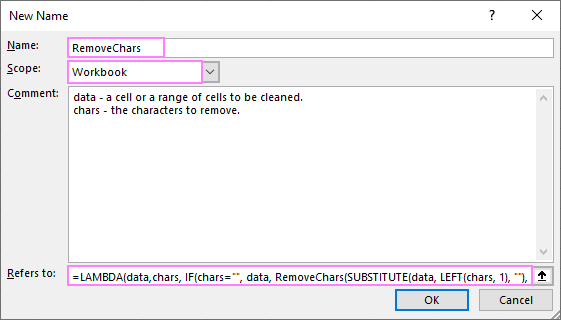## How to use a recursive LAMBDA in Excel

It is the easiest part :) Once your Lambda function gets a name, you can use it just like any other native function.

From the end-user perspective, the syntax of our custom function is as simple as this:

RemoveChars(data, chars)

For example, to clean the data in A2:A10 (data), we type the unwanted characters in D2 (chars), and then enter the below formula in B2:

`=RemoveChars(A2:A10, D2)`

As you probably know, in Excel 356, every formula is a dynamic array formula by nature. So, having the formula entered in just one cell (B2), we immediately get all the results (this behavior is called spilling).If you prefer the traditional "one formula - one cell" behavior, then use a cell reference for data (A2) and lock the chars cell address (\$D\$2) with the \$ sign to prevent it from changing when copying the formula down:

`=RemoveChars(A2, \$D\$2)`

The above formula goes to B2, and then you drag it through B10: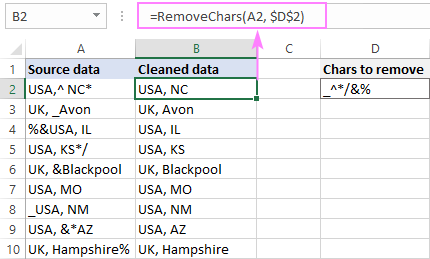Instead of listing the to-be-removed characters in a cell, you can supply them directly to the formula as a text string:

`=RemoveChars(A2:A10, "_^*/&%")`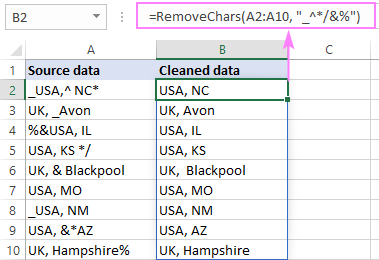Note. Because the SUBSTITUTE function used in the core formula is case-sensitive, our custom function treats uppercase and lowercase letters as different characters. If you want to remove a certain character, say "x", regardless of the letter case, then include both "x" and "X" in the chars string.

## Understanding recursion

The clue to understanding recursive Lambdas is knowing exactly what happens with each iteration. In our example, there are two such things:

• The result from the previous SUBSTITUTE becomes the new data parameter for the next call of RemoveChars, as if we used nested SUBSTITUTE functions.
• The chars string is reduced by one character. You can think of it as a kind of countdown. Once the chars string becomes empty, the iteration process stops, and the formula returns data in its present form as a final result.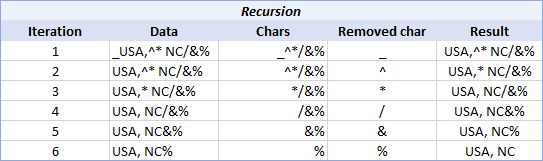## More examples of recursive LAMBDA function

In the below examples, we will look at how you can extend the existing LAMBDA function with new functionality to adjust it for your needs.

### Example 1. Remove unwanted characters and trim extra spaces

Besides various irrelevant characters, your data may also contain excessive spaces. To get rid of them, you can nest RemoveChars inside of TRIM like you would any built-in function:

`=TRIM(RemoveChars(A2:A10, F2))`

To see the effect, please compare the results in columns B and D. In the latter case, not only unwanted characters are removed, but also all leading and trailing spaces, while inner spaces are reduced to a single space character between words: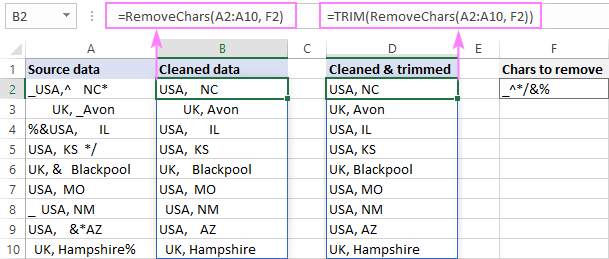If you don't want to bother with nesting every time, you can do it as a one-time setup inside the LAMBDA itself:

`=LAMBDA(data, chars, TRIM(IF(chars<>"", RemoveTrim(SUBSTITUTE(data, LEFT(chars, 1), ""), RIGHT(chars, LEN(chars) -1)), data)))`

Our improved function is named RemoveTrim and it works like a charm:

`=RemoveTrim(A2:A10, D2)`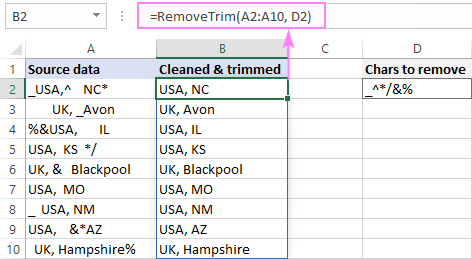### Example 2. Replace multiple characters with the same character

In certain scenarios, it makes sense to replace a few different characters with another character that you specify. In fact, it is what our RemoveChars function actually does - replaces the specified characters with an empty string (""). However, the replacement character is hardcoded whilst we want to define it directly in the formula. To have it done, we just need to add one more parameter, say new_char, to the function.

So, our new function, let's name it ReplaceChars, will have the following syntax:

ReplaceChars(data, chars, new_char)

To transform RemoveChars into ReplaceChars, there are 3 small edits to be made:

• Define the 3rd parameter - new_char.
• Replace the hardcoded empty string ("") with new_char.
• Pass new_char to the ReplaceChars function as the 3rd argument.

In the result, we get yet another useful Lambda to replace multiple characters recursive:

`=LAMBDA(data, chars, new_char, IF(chars<>"", ReplaceChars(SUBSTITUTE(data, LEFT(chars), new_char), RIGHT(chars, LEN(chars)-1), new_char), data))`

For instance, if your supplier suddenly changes their IDs or SKUs formats, you can replace all inappropriate characters (E1) with the appropriate one (E2) using this formula:

`=ReplaceChars(A2:A6, E1, E2)`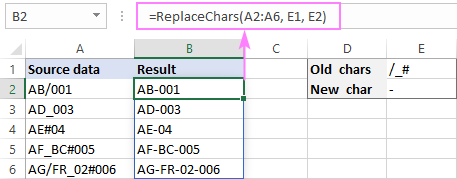### Example 3. Replace multiple values with other values at once

This example is a logical extension of the one before it. This time, we will be replacing entire words (or strings) rather than single characters, and each word will have its own replacement value.

Because the old and new values are going to be placed in separate cells (as shown in the screenshot below), the RIGHT function we used in the previous examples won't work. To loop through the old/new pairs, we need to figure out something else. Hmm, there seems to be a function in Excel to move a specified number of rows and columns from a given cell. Yep, that's OFFSET!

With the main method established, it's no big deal to write the ReplaceAll function:

ReplaceAll(data, old, new)

For data, we are using the SUBSTITUTE function in its basic form simply to replace the old value with the new one:

`SUBSTITUTE(data, old, new)`

To get the old value, we'll start with the topmost cell on the Old list and move 1 row down with each interaction:

`OFFSET(old, 1, 0)`

To get the new value, we'll do exactly the same but, of course, on the New list:

`OFFSET(new, 1, 0)`

Finally, implement the already familiar exit strategy with the help of IF, and your new powerful recursive Lambda is ready for use (just don't forget to name it in the Name Manager :)

`=LAMBDA(data, old, new, IF(old<>"", ReplaceAll(SUBSTITUTE(data, old, new), OFFSET(old, 1, 0), OFFSET(new, 1, 0) ), data))`

With the source data in A2:A10, the old values in column D beginning in D2, and the new values in column E beginning in E2, you can do multiple replacements with this simple formula:

`=ReplaceAll(A2:A10, D2, E2)`

As the result, a single formula in B2 replaces all the abbreviations in A2:A10 with the corresponding full names: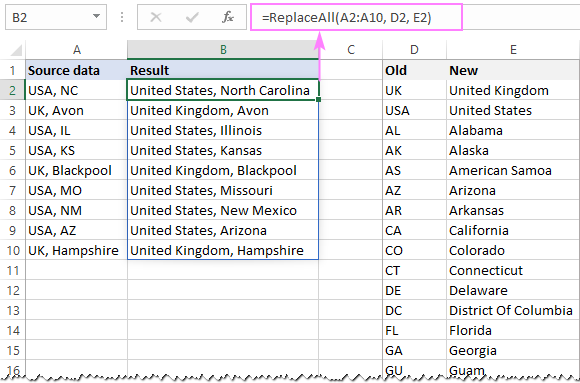## Recursive Lambdas vs. VBA user-defined functions

Advanced Excel users with a programming background may be curious to see how a recursive LAMBDA function correlates with a comparable VBA code. Well, let's take a look.

### Recursive LAMBDA to remove multiple characters

As you understand, this is non-functional pseudocode. We put it in a VBA editor to represent the algorithm in the familiar form to better understand what's going on :)

```=LAMBDA(data, chars,  'declare parameters

IF(

chars<>"",     'check if chars is not empty

RemoveChars(SUBSTITUTE(data, LEFT(chars, 1), ""),   'if TRUE, call RemoveChars
RIGHT(chars, LEN(chars) -1)),

data           'if FALSE, return data and exit

)
)```

### User-defined function to remove multiple characters recursive

And this is how you an analogous user-defined function can be written in VBA:

```Function RemoveCharsRecursive(data As String, chars As String)

If ("" <> chars) Then

data = Replace(data, Left(chars, 1), "")

chars = Right(chars, Len(chars) - 1)

RemoveCharsRecursive = RemoveCharsRecursive(data, chars)

Else

RemoveCharsRecursive = data

End If

End Function```

### User-defined function to remove multiple characters non-recursive

A similar function can also be written using a non-recursive method. In this case, we write RemoveChars as a separate function and call it from within the RemoveCharsNonRecursive function when the chars string is not empty.

```Function RemoveCharsNonRecursive(data As String, chars As String)

While "" <> chars

data = RemoveChars(data, chars)

chars = Right(chars, Len(chars) - 1)

Wend

RemoveCharsNonRecursive = data

End Function

Function RemoveChars(data As String, chars As String)

RemoveChars = Replace(data, Left(chars, 1), "")

End Function```

The same task can be accomplished in a different way. You can iterate through the exclusion characters from 1 to Len(chars) and replace the chars found in data with an empty string. The MID function is used to extract each single character from the chars string one-by-one.

```Function RemoveCharsNonRecursive2(data As String, chars As String)

For Index = 1 To Len(chars)

data = Replace(data, Mid(chars, Index, 1), "")

Next

RemoveCharsNonRecursive2 = data

End Function```

What is the benefit of using Lambdas compared to VBA user-defined functions? First and foremost, they do not require saving workbooks as macro-enabled .xlsm files and save you the trouble of enabling macros on every opening.

Hopefully, this tutorial has helped you get an insight into what a recursive LAMBDA looks like in Excel. I thank you for reading and hope to see you on our blog next week!